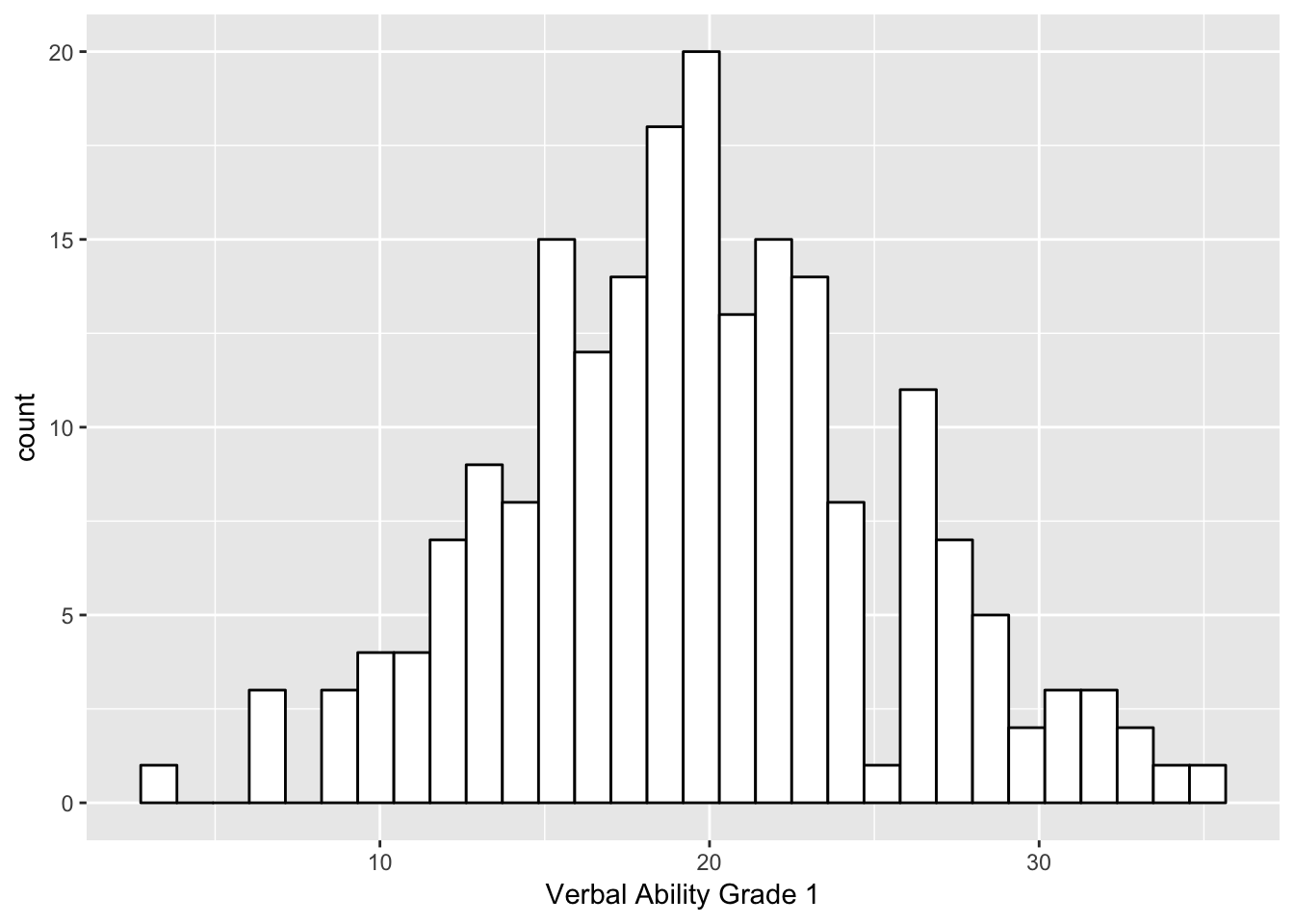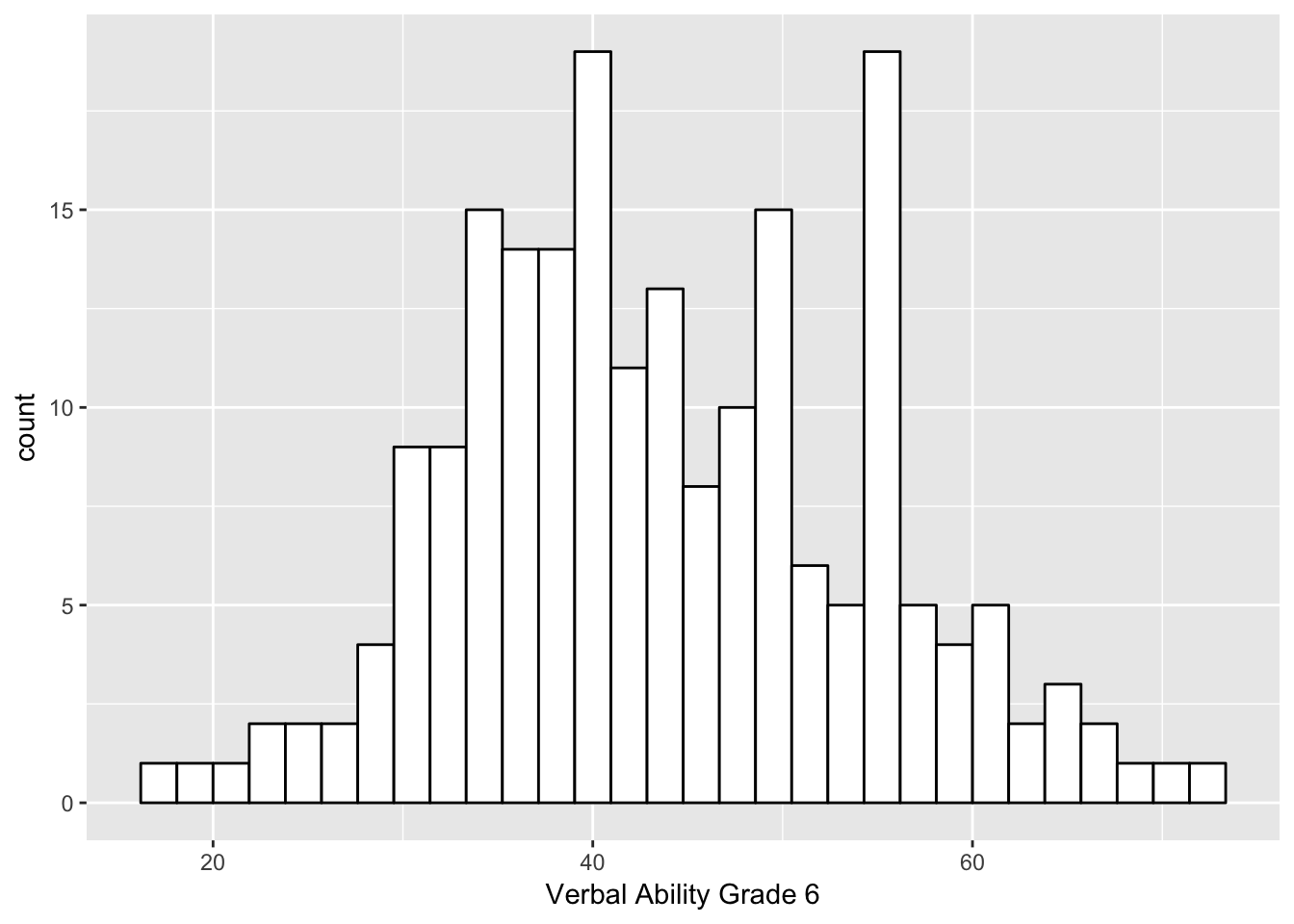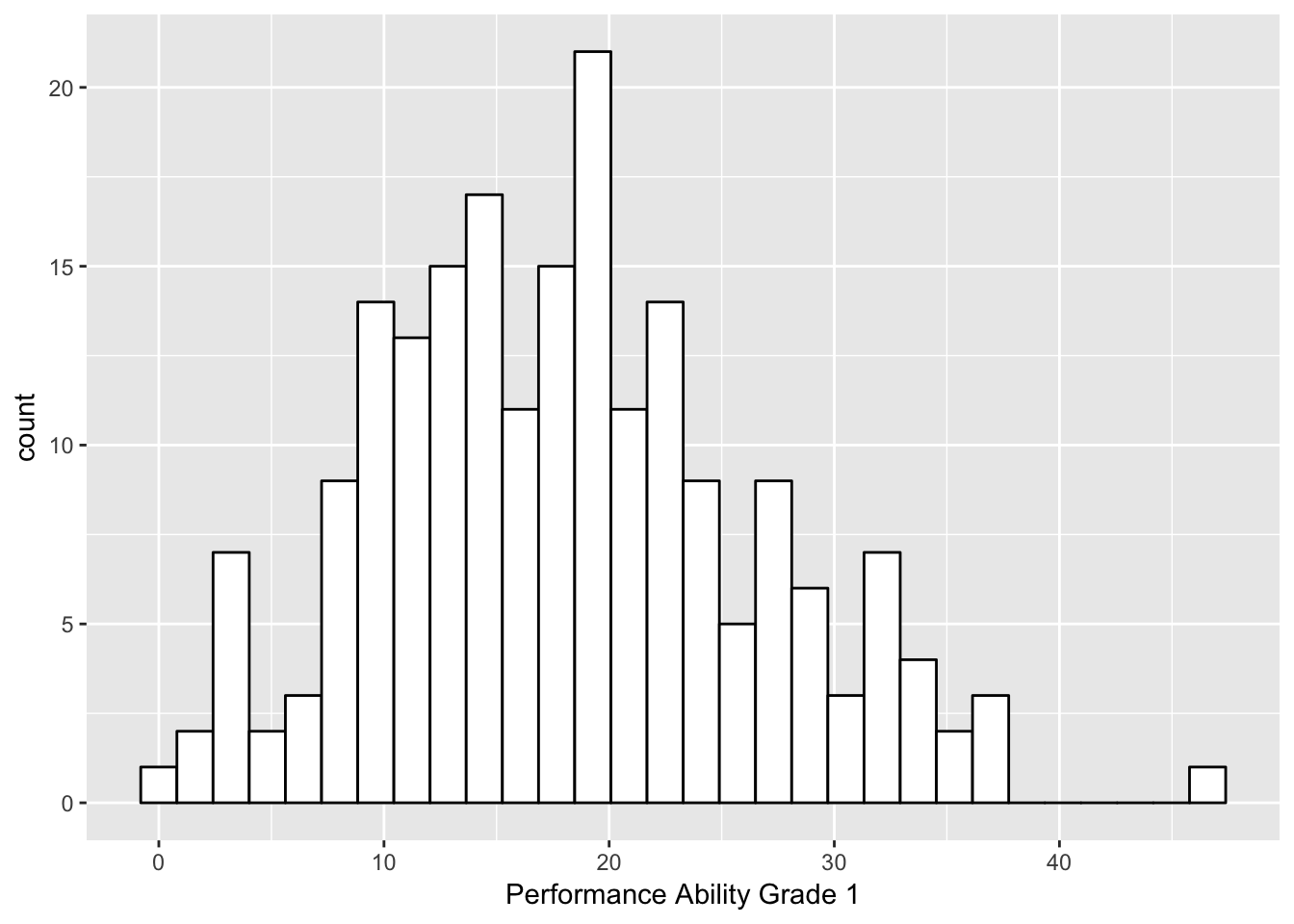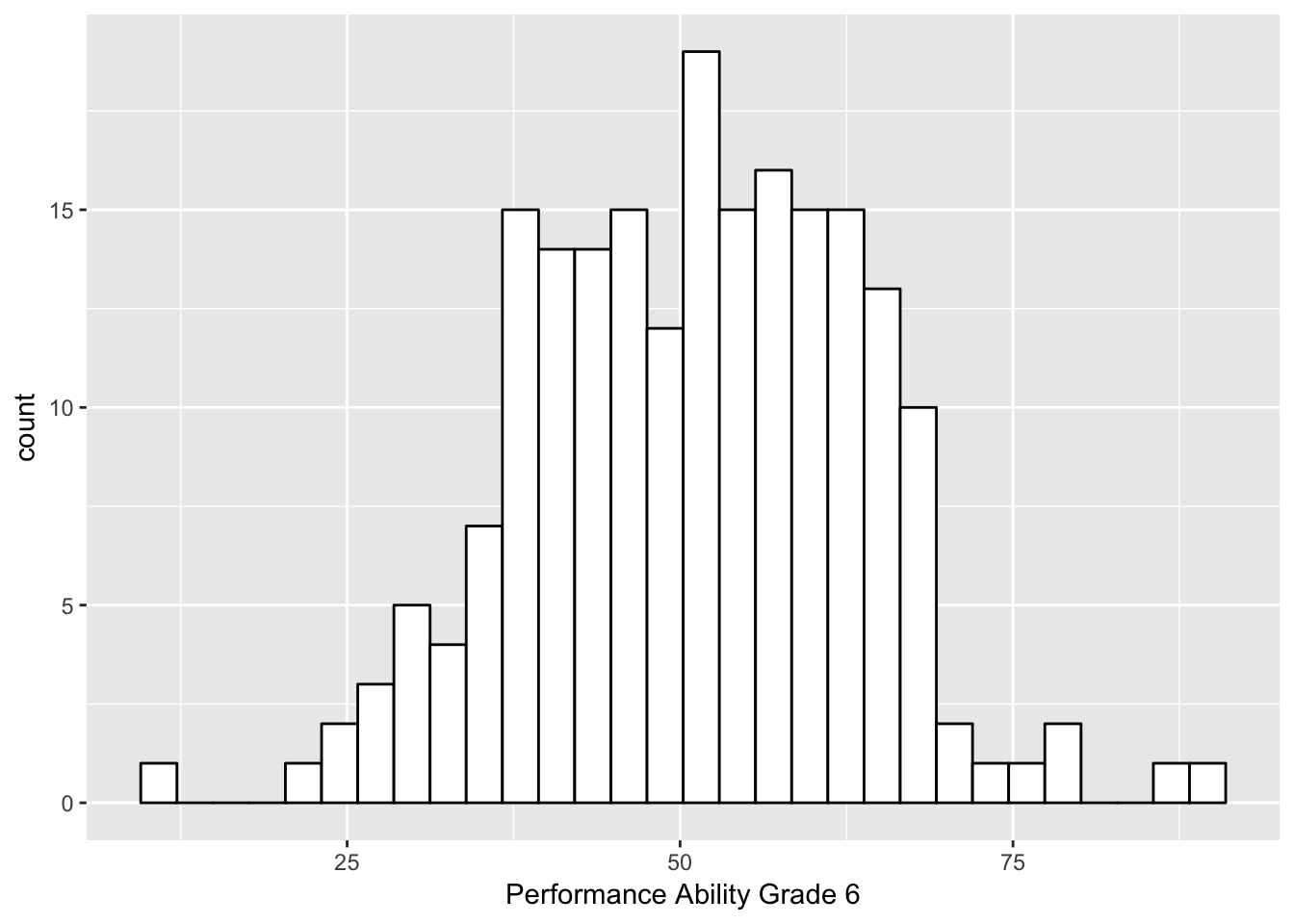# 1 Overview

This tutorial reviews the Actor-Partner Interdependence Model (APIM; Kashy & Kenny, 2000; Kenny, Kashy, & Cook, 2006), which is often used to examine the association (1) between two constructs for two people using cross-sectional data, or (2) between the same construct from two people across two time points.

# 2 Outline

In this tutorial, we’ll cover…

• Useful descriptive statistics for dyadic data
• APIM model using `nlme` package
• Other resources

Before we begin, let’s load the libraries we need.

``````library(ggplot2)
library(nlme)
library(psych)
library(reshape)``````

We’ll be using our trusty WISC data, so we’ll read that data set in.

``````#set filepath for data file
filepath <- "https://quantdev.ssri.psu.edu/sites/qdev/files/wisc3raw_gender.csv"

#read in the .csv file using the url() function

#subset to variables of interest
data <- wisc3raw[, c("id", "verb1", "verb6", "perfo1", "perfo6")]
id verb1 verb6 perfo1 perfo6
1 24.42 55.64 19.84 44.19
2 12.44 37.81 5.90 40.38
3 32.43 50.18 27.64 77.72
4 22.69 44.72 33.16 61.66
5 28.23 70.95 27.64 64.22
6 16.06 39.94 8.45 39.08

We’ll also make a long version of the data set for later use.

``````data_long <- reshape(data = data,
varying = c("verb1", "verb6",
"perfo1", "perfo6"),
idvar = c("id"),
direction = "long", sep="")

#sort for easy viewing - reorder by id and grade

# 3 Problem Definition

In this tutorial, we are going to examine the association between verbal and performance ability using measures from first grade and sixth grade. We are interested in simultaneously examining whether (1) verbal ability in the first grade is predictive of verbal ability in the sixth grade, (2) performance ability in the first grade is predictive of performance ability in the sixth grade, (3) verbal ability in the first grade is predictive of performance ability in the sixth grade, and (4) performance ability in the first grade is predictive of verbal ability in the sixth grade.

When working with people, the above points 1 and 2 are often referred to as “actor effects” and points 3 and 4 are often referred to as “partner effects.”

While this example is not a “traditional” dyad - i.e., two distinguishable people - the analytic processes demonstrated here are applicable to the examination of any bivariate relationship.

# 4 Descriptive Statistics for Dyadic Data

Before we run our models, it is often useful to get more familiar with the data via plotting and descriptive statistics.

Let’s begin with descriptive statistics of our four variables of interest: first grade verbal and performance ability, and sixth grade verbal and performance ability.

``describe(data\$verb1)``
vars n mean sd median trimmed mad min max range skew kurtosis se
1 204 19.58505 5.807695 19.335 19.49768 5.41149 3.33 35.15 31.82 0.1301071 -0.0528376 0.40662
``describe(data\$verb6)``
vars n mean sd median trimmed mad min max range skew kurtosis se
1 204 43.7499 10.66505 42.545 43.4561 11.29741 17.35 72.59 55.24 0.2356459 -0.360521 0.7467029
``describe(data\$perfo1)``
vars n mean sd median trimmed mad min max range skew kurtosis se
1 204 17.97706 8.349782 17.66 17.69116 8.295147 0 46.58 46.58 0.3510232 -0.1089726 0.5846017
``describe(data\$perfo6)``
vars n mean sd median trimmed mad min max range skew kurtosis se
1 204 50.93162 12.47987 51.765 51.07073 13.26927 10.26 89.01 78.75 -0.0637532 0.1836101 0.873766

We can see that both the mean and standard deviation of verbal and performance ability increase from first to sixth grade. While this is worth noting, the APIM will not be examining changes in mean differences of verbal and performance ability.

And we’ll plot the distributions of each of these variables as well.

``````ggplot(data = data, aes(x = verb1)) +
geom_histogram(fill = "white", color = "black") +
labs(x = "Verbal Ability Grade 1") ````````````ggplot(data = data, aes(x = verb6)) +
geom_histogram(fill = "white", color = "black") +
labs(x = "Verbal Ability Grade 6") ````````````ggplot(data = data, aes(x = perfo1)) +
geom_histogram(fill = "white", color = "black") +
labs(x = "Performance Ability Grade 1") ````````````ggplot(data = data, aes(x = perfo6)) +
geom_histogram(fill = "white", color = "black") +
labs(x = "Performance Ability Grade 6") ``````Next, let’s examine the association (i.e., rank order stability) among each variable of interest using correlations and a plot.

``````#correlations
cor(data[, 2:5])``````
``````##            verb1     verb6    perfo1    perfo6
## verb1  1.0000000 0.6541040 0.6101379 0.4779672
## verb6  0.6541040 1.0000000 0.6183155 0.6106694
## perfo1 0.6101379 0.6183155 1.0000000 0.6958321
## perfo6 0.4779672 0.6106694 0.6958321 1.0000000``````
``````#plot
pairs.panels(data[, c("verb1", "verb6", "perfo1", "perfo6")])``````